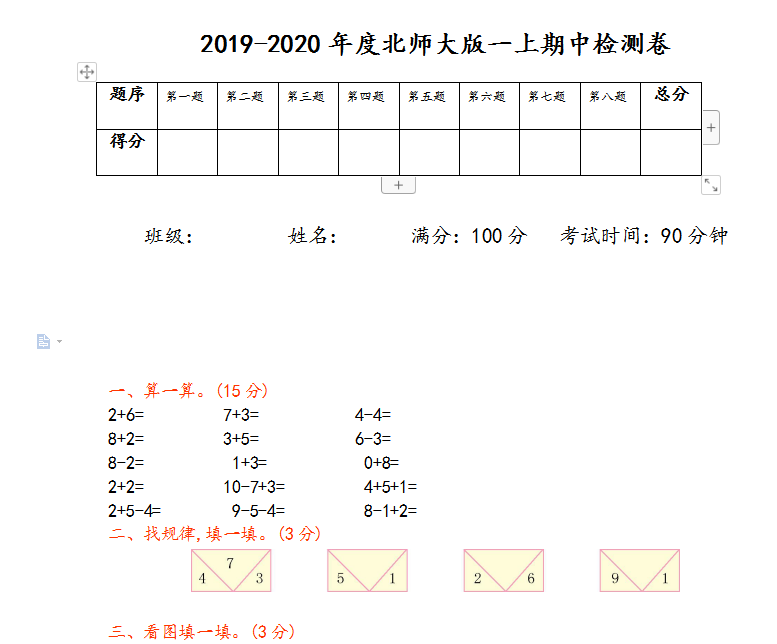2019-2020年度北师大版一上期中检测卷

 题序 第一题 第二题 第三题 第四题 第五题 第六题 第七题 第八题 总分 得分

2+6=　　　　 7+3=　　　   　4-4=

8+2=　　　 　3+5=           6-3=

8-2=        1+3=         0+8=

2+2=         10-7+3=         4+5+1=

2+5-4=        9-5-4=         8-1+2=

1.在高的下面画“�”。(2分)　　　　　　　2.在最长的后面画“�”。(3分)